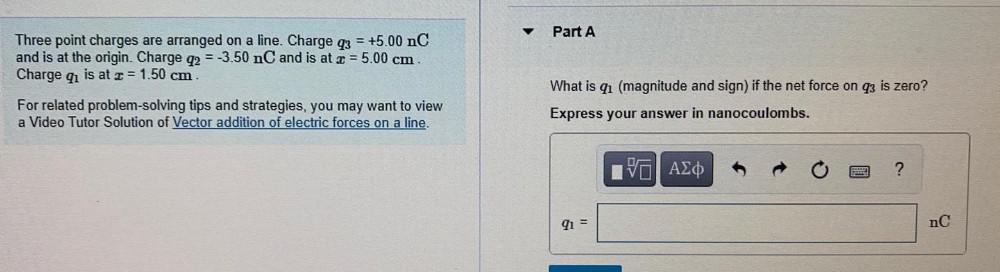Question:

# Part A Three point charges are arranged on a line. Charge 3 = +5.00 nC and is at the origin. Charge q2 = -3.50 nC and is at z =Part A Three point charges are arranged on a line. Charge 3 = +5.00 nC and is at the origin. Charge q2 = -3.50 nC and is at z = 5.00 cm. Charge qı is at z = 1.50 cm For related problem-solving tips and strategies, you may want to view a Video Tutor Solution of Vector addition of electric forces on a line. What is qı (magnitude and sign) if the net force on q3 is zero? Express your answer in nanocoulombs. VALO 91 = nC